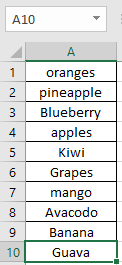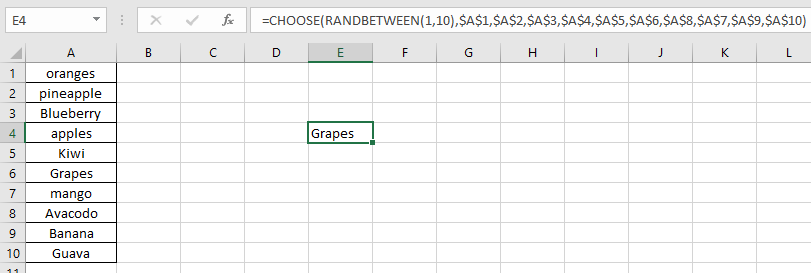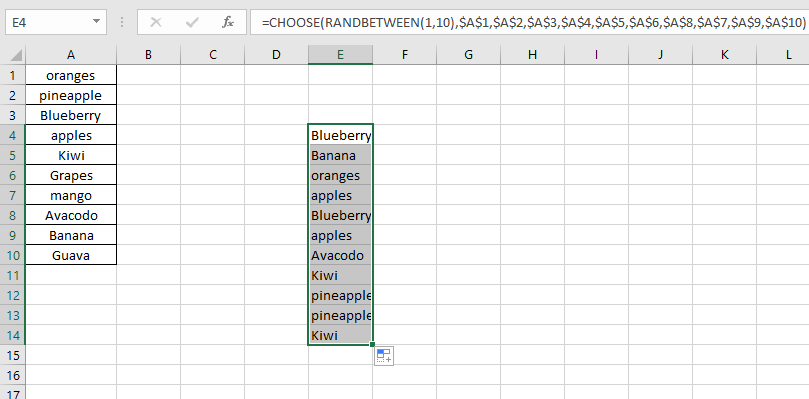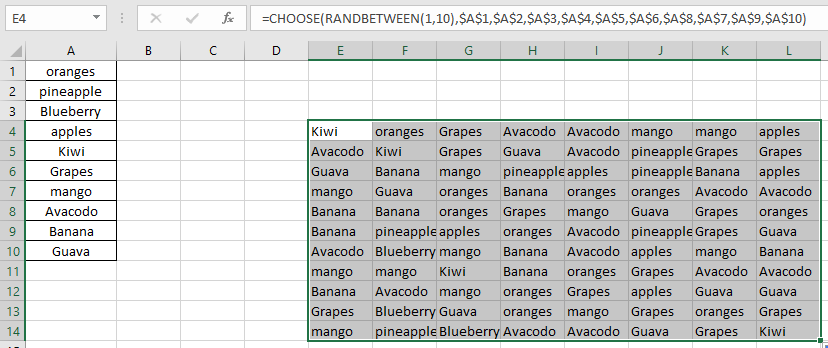# How to Generate Random text values in Excel

In this article, we will learn to generate random text values in  Excel.

1. CHOOSE function
2. RANDBETWEEN function

The CHOOSE function takes values and returns the value corresponding to a particular index_num.

RANDBETWEEN function returns random numbers between any two numbers.

As we know a RANDBETWEEN function returns a number between the two given numbers. So the CHOOSE function will take that number and returns the text value corresponding to the number. Hence Excel returns random text from a list.

Use the formula:

= CHOOSE ( RANDBETWEEN ( 1 , 3) , "Value1" , "Value2" , "Value3" )

1 will return Value1
2 will returns Value2 and so on.

Let's test this formula via running it on an example

Here we have the names of fruits in the A1 to A10 cells.Now we can generate as many numbers of these names as we need.
Use the formula:

= CHOOSE ( RANDBETWEEN ( 1, 10 ), \$A\$1,\$A\$2,\$A\$3,\$A\$4,\$A\$5,\$A\$6,\$A\$8,\$A\$7,\$A\$9,\$A\$10)

\$A\$1 : this is fixed value at A1 cell (\$ sign fixes the cell in the formula)Use the drag down option to generate names.Use the drag right option to generate names.As you can see in the above snapshot the outcomes of the formula.

Notes :

1. The RANDBETWEEN function returns an error, if the first number argument ( bottom ) is larger than the second number argument ( top ).
2. The RANDBETWEEN function returns error, if the argument to the function is non - numeric.
3. The CHOOSE function returns #VALUE! Error if the value argument to the function is given as array.
4. The CHOOSE function returns error if the returned number by the RANDBETWEEN function exceeds the number of values.

Hope this article about how to generate random text values in Excel is explanatory. Find more articles on TEXT functions here. Please share your query below in the comment box. We will assist you.

Related Articles

Excel Random Selection: How to Get Random Samples From a Dataset

Get Random numbers between two numbers.

Get Random number From Fixed Options

Generate Random Phone Numbers

How to use the CHOOSE Function in Excel

How to use the RANDBETWEEN Function in Excel

Popular Articles

50 Excel Shortcut to Increase Your Productivity

Edit a dropdown list

Absolute reference in Excel

If with conditional formatting

If with wildcards

Vlookup by date

Convert Inches To Feet and Inches in Excel 2016

Join first and last name in excel

Count cells which match either A or B

Terms and Conditions of use

The applications/code on this site are distributed as is and without warranties or liability. In no event shall the owner of the copyrights, or the authors of the applications/code be liable for any loss of profit, any problems or any damage resulting from the use or evaluation of the applications/code.